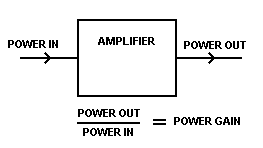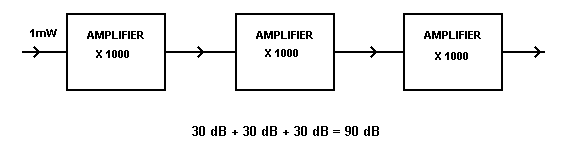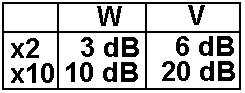Home > Electronics Tutorials > Bels Decibels and DB Tutorial

# Bels Decibels and DB Tutorial

Bels are a means of comparing two powers or two voltages.

For example, comparing the power input to an amplifier with the power output as in the diagram below.

The ratio of power in, to power out, is the power gain.In the next diagram, we have three stages of amplification.

To find the overall power gain, we multiply the individual gains.

10 X 6.5 X 9.2 = ?

Multiplying is not friendly and also large numbers can result.If we find the log of the power ratio, the answer is in Bels.

To do this, we work out the power ratio and then look up the log of this value in log tables.Since Bels are too big , we work in decibels (dBs) as shown below.One advantage of decibels are that the numbers are usually more convenient.

Below, the overall gain is 1,000,000,000 or +90 dBs.

A gain of 1000 is +30 dB.

To find the overall gain, individual gains are added.

A - sign would indicate an attenuation.If we are using voltage ratios then use the following formula.The ear has a logarithmic response.

To make your amplifier sound twice as loud you must boost the power by 10 times. (+10 dB)

If you only double the power (+3 dB), then you will only just be able to detect the increase in volume.

Other points: there is always a ratio, such as the noise of Concorde compared with the noise of rustling leaves.

Sometimes there are standard levels to be compared with, such as 1 mW into 600 ohms for audio, or 1 volt into 75 ohms for video.

10 dBm, for example, is +10 dB relative to 1 mW.

It is not necessary to do calculations, tables are available, giving the dB equivalent of ratios, and vice-versa.

A useful table is shown below.A gain of 400 watts is 2 x 2 x10 x 10 which is 3 dB + 3 dB + 10 dB + 10 dB = +26 dB.

A voltage gain of + 52 db = 20dB + 20 dB + 6 dB + 6 dB which is 10 x 10 x 2 x 2 = a gain of 400 times.